# Machine Learning and Data Science: Linear Regression Part 4

## Linear Regression Part 4: Parameter Optimization by Gradient Descent¶

Optimizing parameters or “weights” to minimize a Cost Function is one of the fundamental problems in Machine Learning and AI. In this post we are going to look at two methods of finding these optimal parameters for the cost function of our linear regression model.

For this post the method will be simple gradient descent. This presents core ideas that are essential to understanding how machine learning algorithms work. It is only one of many approaches to optimization but the idea of algorithmically minimizing a function with respect to a set of parameters is fundamental. There are well established optimization methods and programs that would be more appropriate for a “real world” setting. However, if you understand gradient descent you understand a lot!

In the post following find optimal parameters will be exact solution of the matrix equations. Linear Regression is solvable using Linear Algebra. Most problems are in general not “solvable” and approximation methods are needed. However, since our cost function is quadratic or “second order” ( a sum of squares ) it will have a linear gradient. [ A gradient is a vector of derivatives ]. The idea of vectorizing a problem is very powerful. It can make implementing a program much easier and more efficient. We will do that in the next post.

I am going to start introducing vector notation in this post. Vectors and Matrices can be thought of as arrays (tables) of “things”( symbols, variables, numbers ). It will become more important … Wikipedia has a good page on Matrices).

The blog posts for Part 1, Part 2 and Part 3 provide background and motivation.

Keep in mind that our simple example of linear regression with 1 feature variable $x$ can be expanded to many variables (dimensions) $x_i$. We could have 10s, 100s or 1000s of feature variables $x_i$ and we would then have similar numbers of parameters to optimize. We could also have complicated non-linear terms for our features $x_i$ like $x^2, x^3, x_1x_2, \ln(x), e^{-x}, 1/x, …$. As long as the parameters $\{a_0, a_1, a_2, … a_n \}$ are linear in our cost function we can use linear regression i.e. multi-variate linear regression. It can be very powerful!

### Notation review¶

• Model function — $h_a(x) = a_0 + a_1x$
• Cost Function — $J(a_0, a_1) = \frac{1}{2m} \sum_{i=1}^{m}(h_a(x^{(i)}) – y^{(i)})^2$ $= \frac{1}{2m} \sum_{i=1}^{m}((a_0 + a_1x^{(i)}) – y^{(i)})^2$
• $a = \{a_0, a_1\}$ — is a set of parameters for the function $h_a(x)$ and the variables for $J(a_0,a_1)$. We want to find the “best” parameters.
• $x$ — is our input variable (i.e. the size of a house in square feet ).
• $y$ — is the output variable (selling price of the house). $h_a(x)$ is an approximation of $y$, $h_a(x) \approx y$.
• Data representation — Our data is represented as pairs of values $(x^{(i)}, y^{(i)})$.

• The superscript $(i)$ is referring to the $i^{th}$ data pair in our training set.

• $m$ will be the number of data points.

A gradient is a “vector” (an ordered list) of derivatives (partial-derivatives). For a simple 1-d curve a derivative at some point is the “slope” of a line that tells you the rate of change of “steepness” at that point. Our model function is a line and the derivative with respect to $x$ is just $a_1$, the slope of the line. Our cost function, $J$ is a function of 2 variables, $\{a_0, a_1\}$ and is parabolic i.e. 2nd order, has “power” 2. [In the last post we looked at graphs of $J$.]

gradient descent optimization is also know as “steepest descents”

### The Gradient of the Cost Function $J(a_0,a_1)$¶

Lets derive the gradient of $J$. This will be finding the partial derivatives of $J$ with respect to the variables $a_0, a_1$.

$$J(a_0, a_1) = \frac{1}{2m} \sum_{i=1}^{m}((a_0 + a_1x^{(i)}) – y^{(i)})^2$$

The gradient “operation” is given by the symbol “nabla”, $\nabla$. The gradient is a column vector of partial derivatives.

$$\nabla J(a_0,a_1) = \begin{bmatrix} \left(\frac{\partial J}{\partial a_0}\right) \\ \left( \frac{\partial J}{\partial a_1}\right) \end{bmatrix}$$

If you know just a little bit of calculus and realize that the derivative of a sum is a sum of derivatives, then it’s easy to see that the partial derivatives are,

$$\frac{\partial J}{\partial a_0} = \frac{1}{m} \sum_{i=1}^{m}((a_0 + a_1x^{(i)}) – y^{(i)}) \ = \frac{1}{m} \sum_{i=1}^{m}(h_a(x^{(i)}) – y^{(i)})$$$$\frac{\partial J}{\partial a_1} = \frac{1}{m} \sum_{i=1}^{m}((a_0 + a_1x^{i}) – y^{(i)})x^{(i)} = \frac{1}{m} \sum_{i=1}^{m}(h_a(x^{(i)}) – y^{(i)})x^{(i)}$$

The gradient descent algorithm is very simple. Iterate (repeat) k steps in the direction of the negative of the gradient until the cost function stops getting smaller or the size of the gradient becomes zero.

$$(a_0)_{k+1} \gets (a_0)_k – \alpha \frac{1}{m} \sum_{i=1}^{m}(h_a(x^{(i)}) – y^{(i)})$$$$(a_1)_{k+1} \gets (a_1)_k – \alpha \frac{1}{m} \sum_{i=1}^{m}(h_a(x^{(i)}) – y^{(i)})x^{(i)}$$

$\alpha$ is the “step size” i.e. how far we are going to go in the (-) direction of the gradient.

It looks a little cleaner in vector form,

$$a = \begin{bmatrix} a_0 \\ a_1 \end{bmatrix}$$$$a_{k+1} \gets a_k – \alpha \nabla J(a_k)$$

The $k=1$ iteration starts with some guess for the values of $a$. Then you update $a$ and repeat until the cost function $J$ stops getting smaller or the gradient becomes zero. The $\gets$ just means “update from”. Note that the parameters $a_0$ and $a_1$ are both updated each iteration.

Imagine this: Say you are on a hill and you want to walk down. You can look around from where you are and find the steepest direction that goes downhill. That’s (-) gradient. Take a step in that direction. That’s step size $\alpha$. Then you repeat that from your new location. You will eventually get to the bottom of the hill. — That’s gradient descent.

#### The step size $\alpha$¶

The step size is a parameter of the algorithm. In Machine Learning understanding what the parameters of the methods you are using do can be as important as understanding the parameters of your model. It’s an important part of the craft. If you take very small steps the algorithm may eventually find the minimum but may take a long time. If your steps are too large you may not get to the bottom because you keep stepping over the minimum.

I’ll do a simple example problem of finding the minimum of $f(x)=x^2$ with gradient descent so you can see this.

[ The gradient $\nabla f(x)$ is just the derivative $\frac{df}{dx} = 2x$ so the gradient descent $x$ update is $x_{k+1} \gets x_k – \alpha 2x_k$ ]

First we’ll load some Python modules and define the functions above.

In :
import pandas as pd  # data handeling
import numpy as np   # numeriacal computing
import matplotlib.pyplot as plt  # plotting core
import seaborn as sns  # higher level plotting tools
%matplotlib inline
sns.set()

In :
# simple function to demo step size
def f(x) : # A parabola
f = x**2
return f
def Df(x) : # The derivative (gradient)
Df = 2*x
return Df
def xp1(x,alpha) : # update
xp1 = x - alpha * Df(x)
return xp1


Here is what happens if your step size is too small. It’s slow to converge.

In :
def plot_steps( guess, alpha, nsteps) :
fig, ax = plt.subplots()
x = np.linspace(-3,3,100)
ax.plot(x, f(x))
x = guess
ax.plot(x,f(x), 'o', label='start x=%.2f' %x )
for i in range(nsteps):
xold = x
x = xp1(x,alpha)
ax.plot(x,f(x), 'o', label='x = %.2f' %x)
ax.plot([xold,x],[f(xold),f(x)], '-')
plt.legend();

In :
plot_steps( -2.5, 0.02, 10 )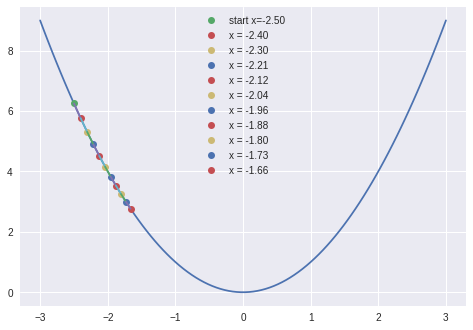If the step size is too big it may overshoot the minimum and oscillate without getting to the minimum.

In :
plot_steps( -2.5, 0.9, 10 )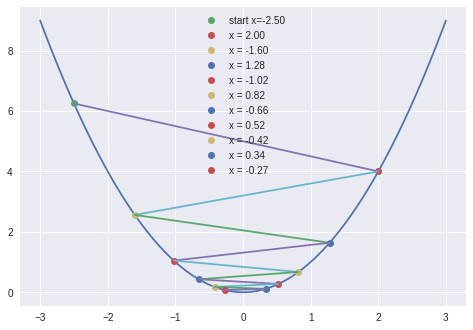If it’s beyond a critical size it will “blow up” and start going up hill to infinity!

In :
plot_steps( -2.5, 1.05, 10 )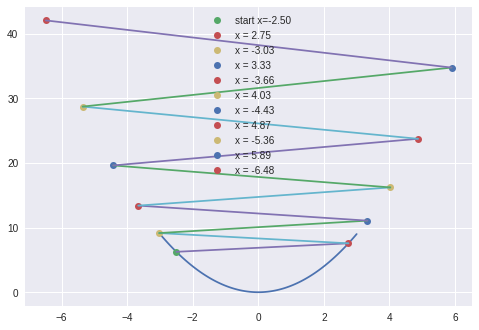Here’s a quiz for you 🙂 If you set the step size $\alpha$ to 0.5 how many steps will it take to find the minimum? [Hint: think about where the minimum is i.e. $x=0$ then look at the gradient descent update formula $x_{k+1} \gets x_k – \alpha 2x_k$ — You could cheat too and download the notebook and make the plot

The step size problem is not too bad if you have your data scaled well (preconditioned). More sophisticated optimization algorithms would use something called a “line search” to find a good step size.

It is important to understand the algorithms you use and know what the algorithm parameters do. If you just blindly use some code you may not get very good results and waste a lot of time.

## Gradient Descent parameter optimization for 98039 zipcode housing data.¶

OK, lets do this with our Housing “price” vs “sqft_living” data. We will iterate through gradient descent and look at the results as we go.

First get the data loaded. It’s on my github page with the notebooks

In :
df_98039 = pd.read_csv("df_98039.csv")
x = df_98039['sqft_living']
y = df_98039['price']
m = len(x)  # Number of data pairs


Now define functions for the formulas of the model, cost function, gradient and parameter updates.

In :
def h(x,a0,a1) :  # The model function h
h = a0 + a1*x
return h

def J(a0, a1, x, y, m):  # The Cost function J
J = 0
for i in range(m):
J += ( h(x[i],a0,a1) - y[i] )**2
return J/(2.0*m)

DJa0 = 0; DJa1 = 0;
for i in range(m):
DJa0 += (h(x[i],a0,a1) - y[i])
DJa1 += (h(x[i],a0,a1) - y[i])*x[i]

def ap1(a0, a1, gJ, alpha) :  # The update to the parameter vector a, ap1 (a plus 1)
# gJ is the gradient vector and alpha is the step length
a0p1 = a0 - alpha * gJ
a1p1 = a1 - alpha * gJ
ap1 = [a0p1, a1p1]
return ap1


### Feature scaling and data transformations¶

Our data is ‘prices’ in the millions and ‘sqft’ in the thousands. Those are big numbers. Remember that the cost function $J(a_0,a_1)$ is a sum of squares over the data set. If we leave the numbers as they are the algorithm will be numerically unstable and could possible “overflow” a.k.a blow up.

Optimization has a habit of bring out the worst behavior in functions so we should consider scaling the data before doing the gradient descent.

As a first try at scaling lets get the data down to similar scales of small numbers. We can divide the price by 1000000 and the sqft by 1000.

In :
plt.plot(x,y, 'o', color='g', label='raw training data'); plt.legend(fontsize=20);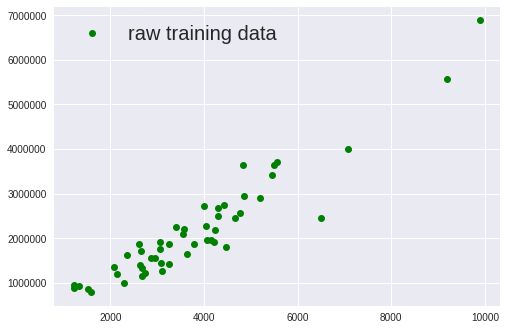In :
plt.plot(x/1000,y/1000000, 'o', color='g', label='Simple scaled training data'); plt.legend(fontsize=20);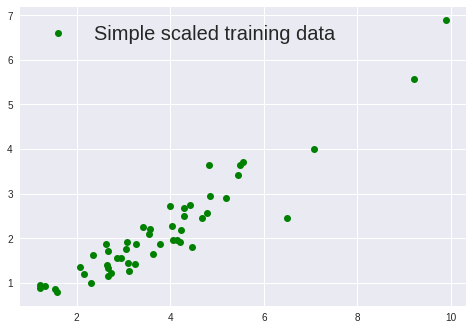That looks more reasonable. We’ll do a gradient descent with this scaling and make a contour plot of the progress. Note, without doing this scaling I couldn’t even get the plot to display or the optimization to start!

In :
fig, ax = plt.subplots()
# setup the contour axis
p0 = np.linspace(-8,8, 500)
p1 = np.linspace(-3,3, 500)
pa0, pa1 = np.meshgrid(p0, p1)

# plot the Cost function J
ax.contour(pa0,pa1,J(pa0,pa1,x/1000,y/1000000,m=len(x)) , colors='C0', levels=[i for i in np.arange(0,80,5)])

# starting point
a0 = 6; a1 = 2.2
ax.plot(a0,a1, 'o')

# do the gradient descent loop and plot the progress
for i in range(300):
a0old, a1old = a0, a1
a0,a1 = ap1(a0,a1, gJ=gradJ(a0,a1,x/1000,y/1000000,m), alpha=0.1 )
#print(a0,a1) # the updated parameters
ax.plot(a0,a1,'o')
ax.plot([a0old,a0],[a1old,a1], '-') # connect the dots

# the values of the parameters after the optimization run
print(a0,a1)

-0.167211102662 0.61403026067